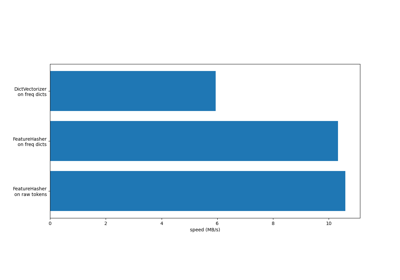sklearn.feature_extraction.FeatureHasher¶

class sklearn.feature_extraction.FeatureHasher(n_features=1048576, input_type=’dict’, dtype=<class ‘numpy.float64’>, alternate_sign=True)[source]

Implements feature hashing, aka the hashing trick.

This class turns sequences of symbolic feature names (strings) into scipy.sparse matrices, using a hash function to compute the matrix column corresponding to a name. The hash function employed is the signed 32-bit version of Murmurhash3.

Feature names of type byte string are used as-is. Unicode strings are converted to UTF-8 first, but no Unicode normalization is done. Feature values must be (finite) numbers.

This class is a low-memory alternative to DictVectorizer and CountVectorizer, intended for large-scale (online) learning and situations where memory is tight, e.g. when running prediction code on embedded devices.

Read more in the User Guide.

Parameters: n_features : integer, optional The number of features (columns) in the output matrices. Small numbers of features are likely to cause hash collisions, but large numbers will cause larger coefficient dimensions in linear learners. input_type : string, optional, default “dict” Either “dict” (the default) to accept dictionaries over (feature_name, value); “pair” to accept pairs of (feature_name, value); or “string” to accept single strings. feature_name should be a string, while value should be a number. In the case of “string”, a value of 1 is implied. The feature_name is hashed to find the appropriate column for the feature. The value’s sign might be flipped in the output (but see non_negative, below). dtype : numpy type, optional, default np.float64 The type of feature values. Passed to scipy.sparse matrix constructors as the dtype argument. Do not set this to bool, np.boolean or any unsigned integer type. alternate_sign : boolean, optional, default True When True, an alternating sign is added to the features as to approximately conserve the inner product in the hashed space even for small n_features. This approach is similar to sparse random projection.

DictVectorizer
vectorizes string-valued features using a hash table.
sklearn.preprocessing.OneHotEncoder
handles nominal/categorical features.

Examples

>>> from sklearn.feature_extraction import FeatureHasher
>>> h = FeatureHasher(n_features=10)
>>> D = [{'dog': 1, 'cat':2, 'elephant':4},{'dog': 2, 'run': 5}]
>>> f = h.transform(D)
>>> f.toarray()
array([[ 0.,  0., -4., -1.,  0.,  0.,  0.,  0.,  0.,  2.],
[ 0.,  0.,  0., -2., -5.,  0.,  0.,  0.,  0.,  0.]])

Methods

 fit(self[, X, y]) No-op. fit_transform(self, X[, y]) Fit to data, then transform it. get_params(self[, deep]) Get parameters for this estimator. set_params(self, \*\*params) Set the parameters of this estimator. transform(self, raw_X) Transform a sequence of instances to a scipy.sparse matrix.
__init__(self, n_features=1048576, input_type=’dict’, dtype=<class ‘numpy.float64’>, alternate_sign=True)[source]
fit(self, X=None, y=None)[source]

No-op.

This method doesn’t do anything. It exists purely for compatibility with the scikit-learn transformer API.

Parameters: X : array-like self : FeatureHasher
fit_transform(self, X, y=None, **fit_params)[source]

Fit to data, then transform it.

Fits transformer to X and y with optional parameters fit_params and returns a transformed version of X.

Parameters: X : numpy array of shape [n_samples, n_features] Training set. y : numpy array of shape [n_samples] Target values. X_new : numpy array of shape [n_samples, n_features_new] Transformed array.
get_params(self, deep=True)[source]

Get parameters for this estimator.

Parameters: deep : boolean, optional If True, will return the parameters for this estimator and contained subobjects that are estimators. params : mapping of string to any Parameter names mapped to their values.
set_params(self, **params)[source]

Set the parameters of this estimator.

The method works on simple estimators as well as on nested objects (such as pipelines). The latter have parameters of the form <component>__<parameter> so that it’s possible to update each component of a nested object.

Returns: self
transform(self, raw_X)[source]

Transform a sequence of instances to a scipy.sparse matrix.

Parameters: raw_X : iterable over iterable over raw features, length = n_samples Samples. Each sample must be iterable an (e.g., a list or tuple) containing/generating feature names (and optionally values, see the input_type constructor argument) which will be hashed. raw_X need not support the len function, so it can be the result of a generator; n_samples is determined on the fly. X : scipy.sparse matrix, shape = (n_samples, self.n_features) Feature matrix, for use with estimators or further transformers.

Examples using sklearn.feature_extraction.FeatureHasher¶FeatureHasher and DictVectorizer Comparison